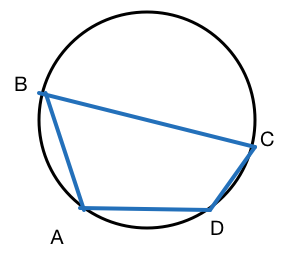# It will all click together

Geometry Level 5Suppose a cyclic quadrilateral $ABCD$ is such that

(i) $AB = AD = 1$,

(ii) $CD = \cos(\angle ABC)$ and

(iii) $\cos(\angle BAD) = -\dfrac{1}{3}.$

Let $R$ be the radius of the circle in which $ABCD$ is inscribed. Find $\lfloor 1000*R \rfloor$.

×